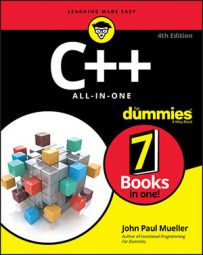##### C++ All-in-One For DummiesClass templates perform the heavy lifting of the template types. You use a class template to define objects of nearly any size. In most cases, you use classes to represent complex objects or to perform tasks ill-suited for function or structure templates.

You normally code classes in a separate file using the name of the class as the filename. The class definition appears in a header file, while the code appears in a code file. To make things a bit easier to understand, this example eschews the normal setup and shows the entire example using a single file.

The example shows a specialized queue implementation. It includes many of the features of a standard queue and then adds a few features to meet special development needs. Queues and other containers tend to contain complex code, but you also need to use them with a variety of data types, making a class template the perfect implementation. The ClassTemplate example here shows the code for this example.

```#include <iostream>
#include <vector>
using namespace std;
template<typename T>
class MyQueue
{
protected:
vector<T> data;
public:
void Remove();
void PrintString();
void PrintInt();
bool IsEmpty();
};
template<typename T>
{
data.push_back(input);
}
template<typename T>
void MyQueue<T>::Remove()
{
data.erase(data.begin());
}
template<typename T>
void MyQueue<T>::PrintString()
{
vector<string>::iterator PrintIt = data.begin();
while (PrintIt != data.end())
{
cout << *PrintIt << endl;
PrintIt++;
}
}
template<typename T>
void MyQueue<T>::PrintInt()
{
vector<int>::iterator PrintIt = data.begin();
while (PrintIt != data.end())
{
cout << *PrintIt << endl;
PrintIt++;
}
}
template<typename T>
bool MyQueue<T>::IsEmpty()
{
return data.begin() == data.end();
}
int main()
{
MyQueue<string> StringQueue;
cout << StringQueue.IsEmpty() << endl;
cout << "Printing strings: " << endl;
StringQueue.PrintString();
cout << StringQueue.IsEmpty() << endl;
StringQueue.Remove();
cout << "Printing strings: " << endl;
StringQueue.PrintString();
StringQueue.Remove();
cout << StringQueue.IsEmpty() << endl;
MyQueue<int> IntQueue;
cout << "Printing ints: " << endl;
IntQueue.PrintInt();
return 0;
}```

The example starts with the class MyQueue. Note that data is a vector, not a queue as you might expect. A queue is an adapter — as such, it doesn’t provide support for many of the features found in containers, such as vector — one of which is the use of iterators.

This example uses an iterator for printing, so it relies on a vector, rather than a queue as a starting point. Whenever you create your own specialized version of a common construct, make sure you begin with the right object. Otherwise, you might find the experience of creating the new class frustrating at a minimum, impossible in the worst case.

MyQueue includes the capability to add, remove, and print elements. In addition, you can check whether a queue is empty or full. You have already seen the code for these tasks in other parts of the book.

You might wonder about the code used for printing. The example includes separate methods for printing strings and integers, which might seem counterintuitive. After all, why not simply declare the iterator as follows so that it accepts any data type:

`vector<T>::iterator PrintIt = data.begin();`

The problem is that the iterator requires a specific data type. Consequently, you must declare it as shown. Otherwise you get this unhelpful error message:

`error: expected ';' before 'PrintIt'`

At some point, you want to test this new class using steps similar to those found in main(). The test checks whether the queue actually does detect the empty and filled states, how adding and removing elements works, and whether the print routines work. Here is the output from this example:

```1
Printing strings:
Hello
Goodbye
0
Printing strings:
Goodbye
1
Printing ints:
1
2```